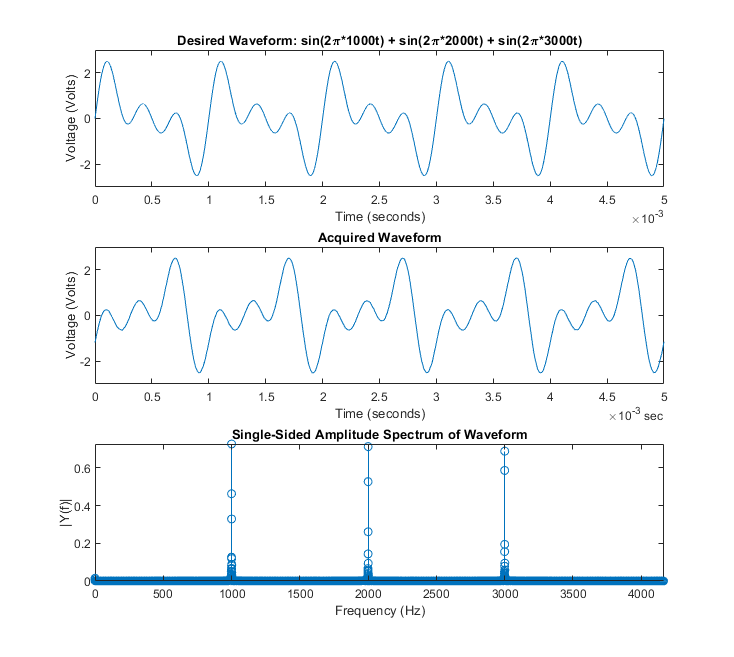Documentation

## Simultaneously Acquire and Generate Arbitrary Periodic Waveforms using Digilent® Analog Discovery™

This example shows how to use the function generator to generate an arbitrary waveform function at a rate of 1 KHz and record data at the same time, using an analog input channel. The output voltage-range of the outgoing signal is -5.0 to +5.0 volts. This example uses the session-based interface with Digilent Analog Discovery hardware.

### Discover Digilent devices

Discover Digilent devices connected to your system using daq.getDevices

daq.getDevices() 
ans = Data acquisition devices: index Vendor Device ID Description ----- ----------- --------- ----------------------------------------------------------------- 1 digilent AD1 Digilent Inc. Analog Discovery Kit Rev. C 2 directsound Audio0 DirectSound Primary Sound Capture Driver 3 directsound Audio1 DirectSound Primary Sound Driver 

### Create a session

s = daq.createSession('digilent') 
s = Data acquisition session using Digilent Inc. hardware: Will run for 1 second (10000 scans) at 10000 scans/second. No channels have been added. 

### Add a function generator channel

Add a function generator channel with device ID AD1 and channel ID 1. Set the waveform type to Arbitrary.

fgenCh = addFunctionGeneratorChannel(s, 'AD1', 1, 'Arbitrary') 
fgenCh = Data acquisition arbitrary waveform generator '1' on device 'AD1': WaveformData: [4096x1 double] Range: -5.0 to +5.0 Volts TerminalConfig: SingleEnded Gain: 1 Offset: 0 Frequency: 4096 WaveformType: Arbitrary FrequencyLimit: [0.0001 25000000.0] Name: '' ID: '1' Device: [1x1 daq.di.DeviceInfo] MeasurementType: 'Voltage' 

### Define the waveform function to be a sum of sinusoids

The function generator produces periodic outputs by repeatedly generating the contents of its buffer (4096 points). A waveform is constructed to fill this buffer without repeating.

buffersize = 4096; len = buffersize + 1; f0 = 1; f1 = 1 * f0; f2 = 2 * f0; f3 = 3 * f0; waveform = sin(linspace(0, 2*pi*f1, len)) + ... sin(linspace(0, 2*pi*f2, len)) + ... sin(linspace(0, 2*pi*f3, len)); A = 5; waveform = A*waveform./max(abs(waveform)); waveform(end) = []; 

### Set the waveform data of the channel

fgenCh.WaveformData = waveform; 

### Set the frequency of the channel to 1 KHz

frate = 1000; fgenCh.Frequency = frate; 

Add an analog input channel with device ID AD1 and channel ID 1. Set the measurement type to Voltage.

aiCh = addAnalogInputChannel(s, 'AD1', 1, 'Voltage') 
aiCh = Data acquisition analog input voltage channel '1' on device 'AD1': Coupling: DC TerminalConfig: Differential Range: -25 to +25 Volts Name: '' ID: '1' Device: [1x1 daq.di.DeviceInfo] MeasurementType: 'Voltage' 

### Define the rate of the acquisition for the session

Acquire data at a higher rate than the highest frequency in the generated waveform (oversample by a factor of 50)

oversamplingratio = 50; nyquistrate = 2 * frate; s.Rate = oversamplingratio * nyquistrate; 

### Define the duration of the acquisition/generation for the session

s.DurationInSeconds = 3; 

### Generate a periodic waveform function and record input simultaneously

[data, timestamps] = startForeground(s); 

### Plot waveform function to be generated by the function generator

% Plot parameters k = 5; period = 1/frate; numperiods = k * period; % Desired waveform wavedesired = repmat(waveform', k, 1); tsamples = linspace(0, numperiods, k * buffersize)'; fig = figure; subplot(311) plot(tsamples, wavedesired); xlabel('Time (seconds)'); ylabel('Voltage (Volts)'); title('Desired Waveform: sin(2\pi*1000t) + sin(2\pi*2000t) + sin(2\pi*3000t)'); axis([0 numperiods -A A]) % Acquired waveform subplot(312) plot(timestamps, data); xlabel('Time (seconds)'); ylabel('Voltage (Volts)'); title('Acquired Waveform'); axis([0 numperiods -A A]) % Frequency-Domain Plot (using fft) L = 2 * oversamplingratio * buffersize; Fs = s.Rate; NFFT = 2^nextpow2(L); Y = fft(data, NFFT)/L; f0 = (Fs/2) * linspace(0, 1, NFFT/2 + 1); plotScaleFactor = 12; plotRange = NFFT / 2; % Plot is symmetric about n/2 plotRange = floor(plotRange / plotScaleFactor); Yplot = Y(1:plotRange); fplot = f0(1:plotRange); % Plot single-sided amplitude spectrum. subplot(313) stem(fplot, 2*abs(Yplot)); title('Single-Sided Amplitude Spectrum of Waveform') xlabel('Frequency (Hz)') ylabel('|Y(f)|') axis tight##### Support평가판 신청